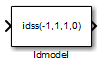# Idmodel

Simulate identified linear model in Simulink software

•Libraries:
System Identification Toolbox / Models

## Description

The Idmodel block simulates the output of an identified linear model using time-domain input data. The model is a state-space (`idss`), linear grey-box (`idgrey`), polynomial (`idpoly`), transfer function (`idtf`), or process (`idproc`) model that you previously estimated or created. For the simulation of state-space and linear grey-box models, you can specify the initial state values. For other linear models, specify initial conditions using an `initialCondition` object. You can also add noise to the simulated output.

## Ports

### Input

expand all

Simulation input data, specified as a scalar for a single-input model. The data must be time-domain data. For multi-input models, specify the input as an Nu-element vector, where Nu is the number of inputs. For example, you can use a Vector Concatenate (Simulink) block to concatenate scalar signals into a vector signal.

Note

Do not use a Bus Creator (Simulink) or Mux (Simulink) block to produce the vector signal.

Data Types: `double`

### Output

expand all

Simulated output from linear model, returned as a scalar for a single-output model and an Ny-element vector for a model with Ny outputs.

Data Types: `double`

## Parameters

expand all

Linear model to be simulated, specified as one of these objects:

This object must be a MATLAB® or model workspace variable for a previously estimated or constructed linear model. The linear model can be continuous-time or discrete-time, and with or without input/output delays.

Initial conditions of the linear model specified as a numeric vector or `initialCondition` object.

• If the linear model specified by Identified model is a state-space (`idss`) or linear grey-box (`idgrey`) model, specify Initial conditions as an Nx-element numeric vector of initial state values, where Nx is the number of states of the model.

• For all other linear models supported by the Idmodel block, specify Initial conditions as an `initialCondition` object. You can also use this object to specify the initial conditions for `idss` and `idgrey` models.

To generate the initial state values or `initialCondition` object used to specify the initial conditions, see Estimate Initial Conditions for Simulating Linear Models.

To denote zero initial conditions, specify Initial conditions as `0` or `[]`.

To reproduce the simulation results that you get in the Model Output plot window in the System Identification app, or from the `compare` command, see Reproduce Command Line or System Identification App Simulation Results in Simulink.

When you select this parameter, the block derives the noise amplitude from the linear model property `model.NoiseVariance`. The software filters random Gaussian white noise with the noise transfer function of the model and adds the resulting noise to the simulated model response. If you want to add the same noise every time you run the Simulink® model, specify the Noise seed(s) parameter.

If you add noise to the simulated output, then to generate the noise at the correct time steps, you must either:

1. Set the sample time of the linear model specified by the Identified model parameter.

2. Specify the Initial conditions parameter as a `initialCondition` object. This object contains model sample time information.

For continuous-time models, the ideal variance of the noise term is infinite. In reality, you see a band-limited noise that accounts for the time constants of the system. You can interpret the resulting simulated output as a response that is filtered using a lowpass filter with a passband that does not distort the dynamics from the input.

The Noise seed(s) property seeds the random number generator such that the block adds the same noise to the simulated output every time you run the Simulink model. For information about using seeds, see `rng`.

For multi-output models, you can use independent noise realizations that generate the outputs with additive noise. Enter a vector of Ny nonnegative integer entries, where Ny is the number of output channels.

For random restarts that vary from one simulation to another, specify Noise seed(s) as `[]`.

#### Dependency

To enable this parameter, select ```Add noise```.

## Version History

Introduced in R2008a